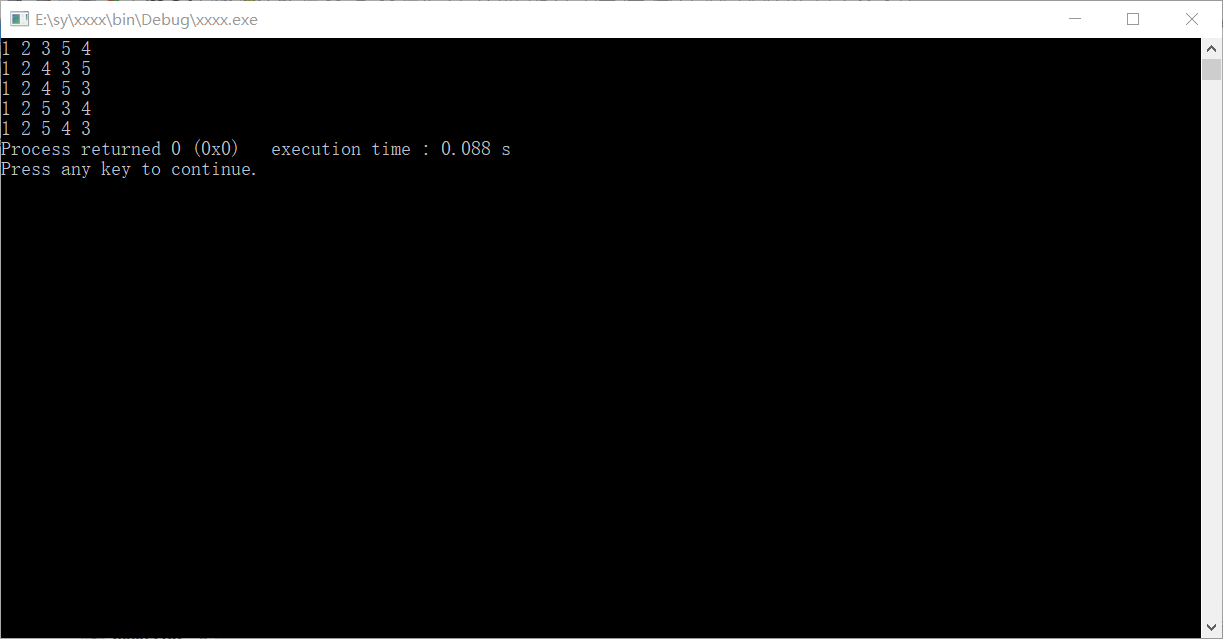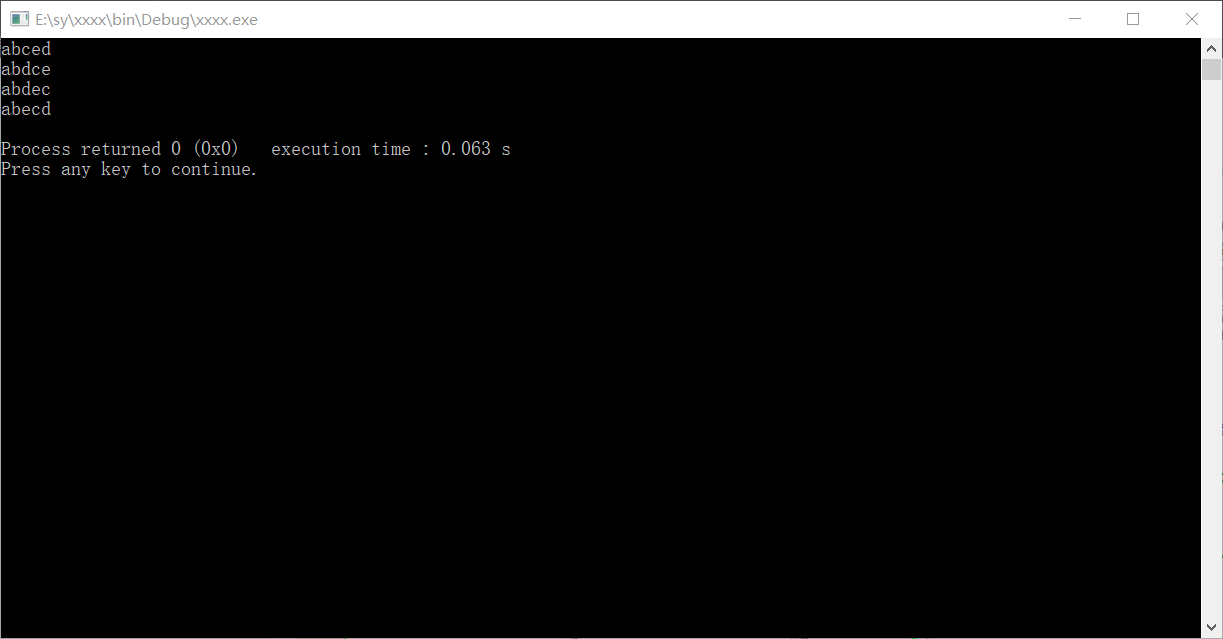• next_permutation函数 next_permutation函数是求全排列的下一位，返回值为bool类型，判断是否有下一位并交换，相对的prev_permutation（）函数是全排列的上一位。 例题：https://ac.nowcoder.com/acm/contest/1086/L...
next_permutation函数
next_permutation函数是求全排列的下一位，返回值为bool类型，判断是否有下一位并交换，相对的prev_permutation（）函数是全排列的上一位。
例题：https://ac.nowcoder.com/acm/contest/1086/L（火星人）
#include <cstdio>
#include <algorithm>
using namespace std ;
const int N = 10005 ;
int a[N] ;
int main(){
int n , m ;
scanf ("%d%d",&n,&m) ;
for (int i = 0 ; i < n ; ++i)
scanf ("%d",&a[i]) ;
while(m --){
//next_permutation(a,a+n) ;
//next_permutation函数的实现
int k = n - 1;
while (a[k - 1] > a[k]) k -- ;
k -- ;
int t = k;
while (t + 1 < n && a[t + 1] > a[k]) t ++ ;
swap(a[t], a[k]);
reverse(a + k + 1, a + n);
}
for (int i = 0 ; i < n ; ++i)
printf ("%d ",a[i]) ;
printf ("\n") ;
return 0  ;
}


展开全文• STL的next_permutation函数可以求出某个特定序列的下一个排列，当然，如果对一个给定序列，排序之后可以轻松求出全排列...... 1 #include<iostream> 2 #include<cstdio> 3 #include<...

STL的next_permutation函数可以求出某个特定序列的下一个排列，当然，如果对一个给定序列，排序之后可以轻松求出全排列......

1 #include<iostream>
2 #include<cstdio>
3 #include<cstring>
4 #include<cmath>
5 #include<algorithm>
6 #include<map>
7 #include<set>
8 #include<vector>
9 #include<sstream>
10 using namespace std;
11 #define ll long long
12 const int inf=99999999;
13 const int mod=1e9+7;
14 //const int maxn=;
15 int num;
16 int main()
17 {
18     ios::sync_with_stdio(false);cin.tie(0);cout.tie(0);
19
20     int n;
21     cin>>n;
22
23     for(int i=0;i<n;i++)
24         cin>>num[i];
25
26     sort(num,num+n);
27
28     cout<<"全排列 ："<<endl;
29     do
30     {
31         for(int i=0;i<n-1;i++)
32             cout<<num[i]<<" ";
33         cout<<num[n-1]<<endl;
34     }
35     while(next_permutation(num,num+n));
36
37     return 0;
38 }

转载于:https://www.cnblogs.com/xwl3109377858/p/10986717.html
展开全文• next_permutation函数 文章目录next_permutation函数前言一、int 类型的next_permutation二、char 类型的next_permutation三，例题总结 前言 输入一个序列，每调用一次，可以求出输入序列的下一个序列，可以遍历...
next_permutation函数

文章目录
next_permutation函数前言一、int 类型的next_permutation二、char 类型的next_permutation三，例题总结

前言
输入一个序列，每调用一次，可以求出输入序列的下一个序列，可以遍历全排列,要包含头文件 与之完全相反的函数还有prev_permutation

一、int 类型的next_permutation

代码如下（示例）：
int main(){
int a;
a=1;a=2;a=3;
do{
cout<<a<<" "<<a<<" "<<a<<endl;
} while (next_permutation(a,a+3)); /*参数3指的是要进行排列的长度
如果存在a之后的排列，就返回true。如果a是最后一个排列没有后继，
返回false，每执行一次，a就变成它的后继*/
}

输出：
1 2 3 1 3 2 2 1 3 2 3 1 3 1 2 3 2 1

如果改成
 while(next_permutation(a,a+2));

则输出： 1 2 3 2 1 3

只对前两个元素进行字典排序 如果改成
 while(next_permutation(a,a+1));

则只输出：1 2 3

若排列本来就是最大的了没有后继，则next_permutation执行后，会对排列进行字典升序排序,相当于循环。
int list={3,2,1};
next_permutation(list,list+3);
cout<<list<<" "<<list<<" "<<list<<endl;

输出: 1 2 3
二、char 类型的next_permutation
代码如下（示例）：
int main(){
char ch;
cin >> ch;
sort(ch, ch + strlen(ch) );
/*该语句对输入的数组进行字典升序排序。如输入9874563102 cout<<ch; 将输出0123456789,这样就能输出全排列了*/
char *first = ch;
char *last = ch + strlen(ch);
do{
cout<< ch << endl;
}while(next_permutation(first, last));
return 0;
}

这样就不必事先知道ch的大小了，是把整个ch字符串全都进行排序

若采用 while(next_permutation(ch,ch+5)); 如果只输入1562，就会产生错误，因为ch中第五个元素指向未知。
若要整个字符串进行排序，参数5指的是数组的长度，不含结束符

三，例题
原题传送门. 题目描述：人类终于登上了火星的土地并且见到了神秘的火星人。
人类和火星人都无法理解对方的语言，但是我们的科学家发明了一种用数字交流的方法。
这种交流方法是这样的，首先，火星人把一个非常大的数字告诉人类科学家，科学家破解这个数字的含义后，再把一个很小的数字加到这个大数上面，把结果告诉火星人，作为人类的回答。
火星人用一种非常简单的方式来表示数字——掰手指。
火星人只有一只手，但这只手上有成千上万的手指，这些手指排成一列，分别编号为1，2，3……。
火星人的任意两根手指都能随意交换位置，他们就是通过这方法计数的。
一个火星人用一个人类的手演示了如何用手指计数。
如果把五根手指——拇指、食指、中指、无名指和小指分别编号为1，2，3，4和5，当它们按正常顺序排列时，形成了5位数12345，当你交换无名指和小指的位置时，会形成5位数12354，当你把五个手指的顺序完全颠倒时，会形成54321，在所有能够形成的120个5位数中，12345最小，它表示1；12354第二小，它表示2；54321最大，它表示120。
下表展示了只有3根手指时能够形成的6个3位数和它们代表的数字：
三位数 123 132 213 231 312 321
代表的数字 1 2 3 4 5 6
现在你有幸成为了第一个和火星人交流的地球人。
一个火星人会让你看他的手指，科学家会告诉你要加上去的很小的数。
你的任务是，把火星人用手指表示的数与科学家告诉你的数相加，并根据相加的结果改变火星人手指的排列顺序。
输入数据保证这个结果不会超出火星人手指能表示的范围。
输入格式 输入包括三行，第一行有一个正整数N，表示火星人手指的数目。
第二行是一个正整数M，表示要加上去的小整数。
下一行是1到N这N个整数的一个排列，用空格隔开，表示火星人手指的排列顺序。
输出格式 输出只有一行，这一行含有N个整数，表示改变后的火星人手指的排列顺序。
每两个相邻的数中间用一个空格分开，不能有多余的空格。
数据范围 1≤N≤10000, 1≤M≤100 输入样例： 5 3 1 2 3 4 5 输出样例： 1 2 4 5 3
1. 直接用next_permutation函数来做
#include<cstdio>
#include<iostream>
#include<cstring>
#include<algorithm>

using namespace std;

const int N=10010;

int n,m;
int a[N];

int main(){
scanf("%d%d",&n,&m);
for(int i=0;i<n;i++)    scanf("%d",&a[i]);
while(m--)  next_permutation(a,a+n);
for(int i=0;i<n;i++)    printf("%d ",a[i]);
return 0;
}

2. 考虑一下next_permutation函数的原理，然后手动实现一遍 对于给定的某个排列，我们想求出比它大的最小的排列。 可以从后往前遍历这个排列，找到第一个可以让排列的字典序变大的位置。
只有当序列单调下降时，它才不存在更大的排列，因此我们要找的位置就是第一次出现 ak−1<akak−1<ak 的位置。
那么此时将 ak−1ak−1 变成比它大的最小数，然后将剩余部分从小到大排序，得到的排列就是比原排列大的最小排列了。
#include <cstdio>
#include <cstring>
#include <iostream>
#include <algorithm>

using namespace std;

const int N = 10010;

int n, m;
int q[N];

int main(){
scanf("%d%d", &n, &m)；
for (int i = 0; i < n; i ++ ) scanf("%d", &q[i]);
while (m -- ){
int k = n - 1;
while (q[k - 1] > q[k]) k -- ;
k -- ;
int t = k;
while (t + 1 < n && q[t + 1] > q[k]) t ++ ;
swap(q[t], q[k]);
reverse(q + k + 1, q + n);
}
for (int i = 0; i < n; i ++ ) printf("%d ", q[i]);
return 0;
}


总结
next_permutation的实现步骤：
从后往前找到第一个a[k]<a[k+1]的位置k在a[k+1…n]中找到大于a[k]的最小数a[t]交换a[k],a[t]，交换后可以发现，a[k+1…n]是单调递减的将a[k+1…n]翻转
展开全文• ## next_permutation函数用法举例

万次阅读 多人点赞 2018-04-28 20:40:00
按照STL文档的描述，next_permutation函数将按字母表顺序生成给定序列的下一个较大的排列，直到整个序列为降序为止。prev_permutation函数与之相反，是生成给定序列的上一个较小的排列。 这是一个求一个排序的下一...
按照STL文档的描述，next_permutation函数将按字母表顺序生成给定序列的下一个较大的排列，直到整个序列为降序为止。prev_permutation函数与之相反，是生成给定序列的上一个较小的排列。
这是一个求一个排序的下一个排列的函数，可以遍历全排列,要包含头文件<algorithm>
使用方法：next_permutation(数组头地址，数组尾地址);若下一个排列存在，则返回真，如果不存在则返回假
若求上一个排列，则用prev_permutation
1.举例
int main()
{
int num = 1,a= {1,2,3,4,5};
while(next_permutation(a,a+5))
{
for(int i=0; i<5; i++)
cout<<a[i]<<" ";
if(num==5)
break;
num++;
cout<<endl;
}
return 0;
}2.字符串（字母数组）

int main()
{
string str = "abcde";
int num = 1;
while(next_permutation(str.begin(),str.end()))
{
num++;
cout<<str<<endl;
if(num==5)
break;
}
return 0;
}在这里补充一道题目HDU - 1716
Ray又对数字的列产生了兴趣：  现有四张卡片，用这四张卡片能排列出很多不同的4位数，要求按从小到大的顺序输出这些4位数。
Input
每组数据占一行，代表四张卡片上的数字（0<=数字<=9），如果四张卡片都是0，则输入结束。
Output
对每组卡片按从小到大的顺序输出所有能由这四张卡片组成的4位数，千位数字相同的在同一行，同一行中每个四位数间用空格分隔。  每组输出数据间空一行，最后一组数据后面没有空行。
Sample Input
1 2 3 4
1 1 2 3
0 1 2 3
0 0 0 0
Sample Output
1234 1243 1324 1342 1423 1432
2134 2143 2314 2341 2413 2431
3124 3142 3214 3241 3412 3421
4123 4132 4213 4231 4312 4321

1123 1132 1213 1231 1312 1321
2113 2131 2311
3112 3121 3211

1023 1032 1203 1230 1302 1320
2013 2031 2103 2130 2301 2310
3012 3021 3102 3120 3201 3210
这里就先对四个数字进行排序，然后用next_permutation函数寻找四个数字的全排列，需要注意的是格式问题，每一行的最后一个数后面没有空格，最后一组数据后面没有空行。我是用一个数组将所有符合条件的四位数存起来，然后进行输出，这样便于控制格式。
AC代码
#include <iostream>
#include <iomanip>
#include <cstdio>
#include <cstring>
#include <algorithm>
#include <vector>
#include <cmath>
using namespace std;
int main()
{
int s,num;
int cnt = 0;
while(scanf("%d%d%d%d",&s,&s,&s,&s) && s + s + s + s)
{
if(cnt++)
printf("\n");
int k = 1;
memset(num,0,sizeof(num));
sort(s,s+4);
for(int i=0; i<4; i++)
num[k] = num[k] * 10 + s[i];
while(next_permutation(s,s+4))
{
k++;
for(int i=0; i<4; i++)
{
num[k] = num[k] * 10 + s[i];
}
//printf("%d\n",num[k]);
}
int t = 1,p;
while(num[t]<1000)
t++;
printf("%d",num[t]);
p = num[t] / 1000;
//printf("%d\n",p);
for(int i=(++t); i<=k; i++)
{
if(((num[i] / 1000) > p))
{
p = num[i] / 1000;
printf("\n%d",num[i]);
}
else
printf(" %d",num[i]);
}
printf("\n");
}
return 0;
}

展开全文...